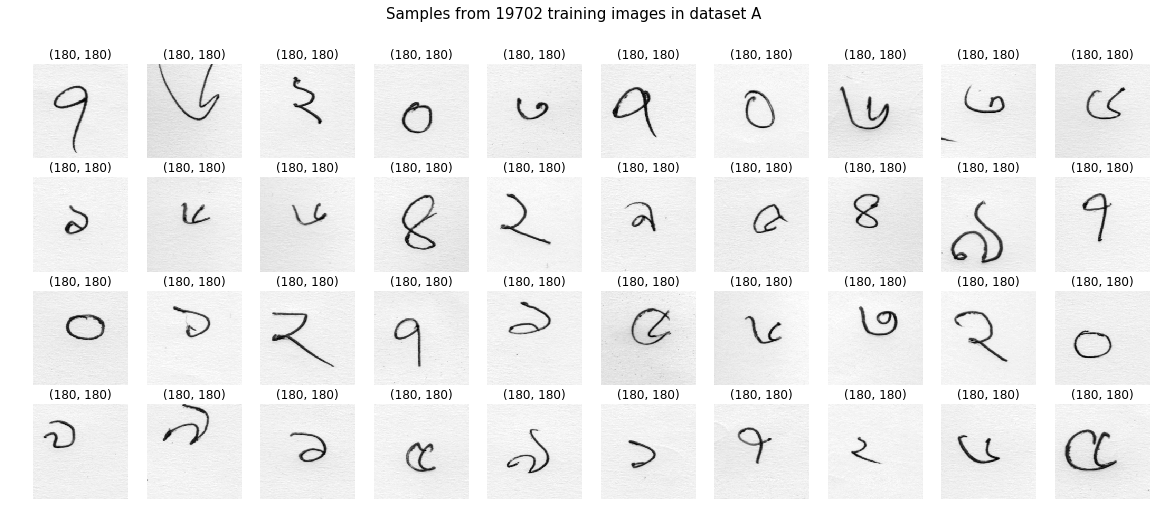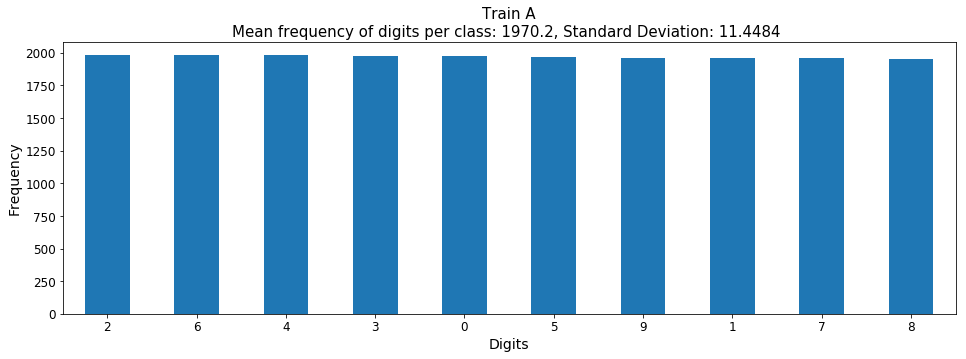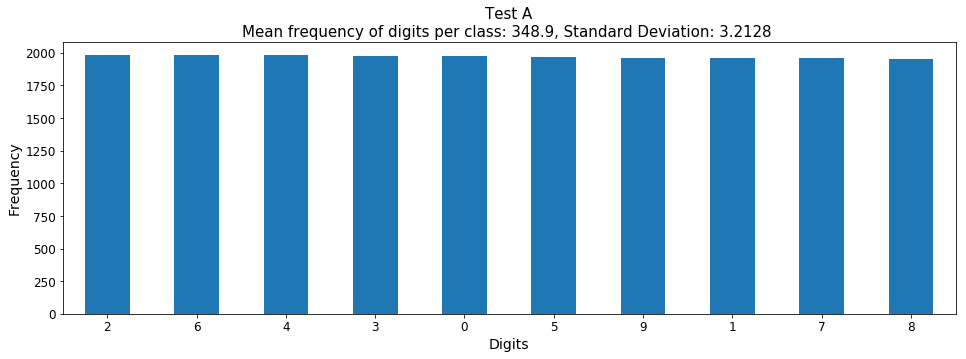# Getting familiar with the data¶

In this notebook, we are going to do some basic exploration of the dataset. We shall observe some samples from each dataset and number of image samples in them. As images are obtained from multiple sources, the quality of the segmentation and the dimension of the images varies a lot. We are going use data analysis toolkit pandas to perform our exploration. If you are not familiar with this library check out their 10 Minutes to pandas short introduction.

In :
# Importing necessary libraries
import numpy as np
import os
import glob
import cv2
import matplotlib.pyplot as plt
import pandas as pd

In :
# Declare fontsize variables which will be used while plotting the data
FS_AXIS_LABEL=14
FS_TITLE=17
FS_TICKS=12
FIG_WIDTH=16


While writing the codes, files and folder was organized in the following way

• OpenBengali
• code
• Final_DB

The code folder contains this jupyter notebook, and the Final_DB folder has the raw image datasets.

In :
project_dir='..'


Let's see what is in the Final_DB folder.

In :
os.listdir(os.path.join(project_dir,'Final_DB'))
# Notice that I am using the os.path.join() function to create the filepaths instead of writing them down explicitly with a
# filepath separator ('\\' for windows '/' for linux). This allows us to run this notebook both in windows and linux
# environment without manually changing the filepath separator

Out:
['testing-a',
'testing-a.csv',
'testing-b',
'testing-b.csv',
'testing-e',
'testing-e.csv',
'training-a',
'training-a.csv',
'training-b',
'training-b.csv',
'training-e',
'training-e.csv']

Our dataset has images from sources A, B, and E. Tha datasets are already split into train and test sets. The labels of each image is saved in the corresponding .csv files. Let's see some samples of the images.

## Setup the path variables¶

All the images have .png extension. We are going get all the filepaths that have .png extensions by using the glob.glob() function

In :
paths_train_a=glob.glob(os.path.join(project_dir,'Final_DB','training-a','*.png'))
paths_train_b=glob.glob(os.path.join(project_dir,'Final_DB','training-b','*.png'))
paths_train_e=glob.glob(os.path.join(project_dir,'Final_DB','training-e','*.png'))
paths_test_a=glob.glob(os.path.join(project_dir,'Final_DB','testing-a','*.png'))
paths_test_b=glob.glob(os.path.join(project_dir,'Final_DB','testing-b','*.png'))
paths_test_e=glob.glob(os.path.join(project_dir,'Final_DB','testing-e','*.png'))
path_label_train_a=os.path.join(project_dir,'Final_DB','training-a.csv')
path_label_train_b=os.path.join(project_dir,'Final_DB','training-b.csv')
path_label_train_e=os.path.join(project_dir,'Final_DB','training-e.csv')
path_label_test_a=os.path.join(project_dir,'Final_DB','testing-a.csv')
path_label_test_b=os.path.join(project_dir,'Final_DB','testing-b.csv')
path_label_test_e=os.path.join(project_dir,'Final_DB','testing-e.csv')


## Some Utility Functions¶

In :
def get_img(path,mode=cv2.IMREAD_GRAYSCALE):
def imshow_group(paths,n_per_row=10):
# plot multiple digits in one figure, by default 10 images are plotted per
n_sample=len(paths)
j=np.ceil(n_sample/n_per_row)
fig=plt.figure(figsize=(20,2*j))
for i, path in enumerate(paths):
img=get_img(path)
plt.subplot(j,n_per_row,i+1)
plt.imshow(img,cmap='gray')
plt.title(img.shape)
plt.axis('off')
return fig
def get_key(path):
# separate the key from the filepath of an image
return path.split(sep=os.sep)[-1]


## Check a few samples from dataset A¶

We are going to randomly choose a few image filepaths from trainnig set A. Then load them in grayscale and plot them.

In :
paths=np.random.choice(paths_train_a,size=40)
fig=imshow_group(paths)
fig.suptitle('Samples from {} training images in dataset A'.format(len(paths_train_a)), fontsize=FS_TITLE)
plt.show()The digits do not fill up the entire image and most of them are not centered.

## Shape statistics of dataset A¶

Next, we are going to observe if images in dataset A have different shapes. To do this, we are going to put the image shapes in a pandas series object and use its .value_counts() attribute to obtain the counts of its unique values.

### Train A¶

In :
shapes_train_a_sr=pd.Series([get_img(path).shape for path in paths_train_a])

In :
shapes_train_a_sr.value_counts()

Out:
(180, 180)    19702
dtype: int64

All the images have a fixed shape of 180 x 180

### Test A¶

In :
shapes_test_a_sr=pd.Series([get_img(path).shape for path in paths_test_a])

In :
shapes_test_a_sr.value_counts()

Out:
(180, 180)    3489
dtype: int64

## Class distribution statistics of dataset A¶

Let's observe the frequency of each digit in dataset A.

### Train A¶

Let's read the .csv file which contains the labels of dataset A. We are using read_csv() function from the pandas library which will return the content of the .csv file in a dataframe.

In :
df_train_a=pd.read_csv(path_label_train_a)
df_train_a.head() # Observe first five rows

Out:
filename original filename scanid digit database name original contributing team database name
0 a00000.png Scan_58_digit_5_num_8.png 58 5 BHDDB Buet_Broncos training-a
1 a00001.png Scan_73_digit_3_num_5.png 73 3 BHDDB Buet_Broncos training-a
2 a00002.png Scan_18_digit_1_num_3.png 18 1 BHDDB Buet_Broncos training-a
3 a00003.png Scan_166_digit_7_num_3.png 166 7 BHDDB Buet_Broncos training-a
4 a00004.png Scan_108_digit_0_num_1.png 108 0 BHDDB Buet_Broncos training-a

Next, we are going to replace the numerical index of the dataframe with the filename column which will give us more convenient access to the dataframe elements.

In :
df_train_a=df_train_a.set_index('filename')
df_train_a.head() # Observe first five rows

Out:
original filename scanid digit database name original contributing team database name
filename
a00000.png Scan_58_digit_5_num_8.png 58 5 BHDDB Buet_Broncos training-a
a00001.png Scan_73_digit_3_num_5.png 73 3 BHDDB Buet_Broncos training-a
a00002.png Scan_18_digit_1_num_3.png 18 1 BHDDB Buet_Broncos training-a
a00003.png Scan_166_digit_7_num_3.png 166 7 BHDDB Buet_Broncos training-a
a00004.png Scan_108_digit_0_num_1.png 108 0 BHDDB Buet_Broncos training-a

As we can see the labels of each digit is located under the digit column. We are going to put the labels of each digit in a series object and use the .value_counts() attribute to get the count of each digit.

In :
labels_train_a_sr=pd.Series([df_train_a.loc[get_key(path)]['digit'] for path in paths_train_a])

In :
labels_train_a_sr_vc=labels_train_a_sr.value_counts()


The pandas series object has a .plot() attribute which is useful to plot the contents of the series. We are going to use it to make a plot of the frequency of each digit.

In :
plt.figure(figsize=(FIG_WIDTH,5))
labels_train_a_sr_vc.plot(kind='bar')
plt.xticks(rotation='horizontal',fontsize=FS_TICKS)
plt.yticks(fontsize=FS_TICKS)
plt.xlabel('Digits', fontsize=FS_AXIS_LABEL)
plt.ylabel('Frequency', fontsize=FS_AXIS_LABEL)
plt.title('Train A\nMean frequency of digits per class: {}, Standard Deviation: {:.4f} '.format(labels_train_a_sr_vc.mean(),labels_train_a_sr_vc.std()),
fontsize=FS_TITLE)
plt.show()### Test A¶

Let's see the class distribution statistics in the test set.

In :
df_test_a=pd.read_csv(path_label_test_a)
df_test_a=df_test_a.set_index('filename')

Out:
original filename scanid digit database name original contributing team database name
filename
a00000.png Scan_178_digit_4_num_2.png 178 4 BHDDB Buet_Broncos test-a
a00001.png Scan_206_digit_9_num_1.png 206 9 BHDDB Buet_Broncos test-a
a00002.png Scan_175_digit_3_num_5.png 175 3 BHDDB Buet_Broncos test-a
a00003.png Scan_96_digit_0_num_2.png 96 0 BHDDB Buet_Broncos test-a
a00004.png Scan_131_digit_4_num_4.png 131 4 BHDDB Buet_Broncos test-a
In :
labels_test_a_sr=pd.Series([df_test_a.loc[get_key(path)]['digit'] for path in paths_test_a])

In :
labels_test_a_sr_vc=labels_test_a_sr.value_counts()
plt.figure(figsize=(FIG_WIDTH,5))
labels_train_a_sr_vc.plot(kind='bar')
plt.xticks(rotation='horizontal',fontsize=FS_TICKS)
plt.yticks(fontsize=FS_TICKS)
plt.xlabel('Digits', fontsize=FS_AXIS_LABEL)
plt.ylabel('Frequency', fontsize=FS_AXIS_LABEL)
plt.title('Test A\nMean frequency of digits per class: {}, Standard Deviation: {:.4f} '.format(labels_test_a_sr_vc.mean(),labels_test_a_sr_vc.std()),
fontsize=FS_TITLE)
plt.show()The digit classes are well balanced both in the test and train set. We are going to repeat the same steps for dataset B and E.

## Check a few samples from dataset B¶

In :
paths=np.random.choice(paths_train_b,size=40)
fig=imshow_group(paths)
fig.suptitle('Samples from {} training images in dataset B'.format(len(paths_train_b)), fontsize=FS_TITLE)
plt.show()